## 4.14.3. True Extent of Datatypes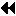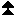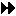Up: New Datatype Manipulation Functions Next: Subarray Datatype Constructor Previous: Extent and Bounds of Datatypes

Suppose we implement gather as a spanning tree implemented on top of point-to-point routines. Since the receive buffer is only valid on the root process, one will need to allocate some temporary space for receiving data on intermediate nodes. However, the datatype extent cannot be used as an estimate of the amount of space that needs to be allocated, if the user has modified the extent using the MPI_UB and MPI_LB values. A new function is provided which returns the true extent of the datatype.

MPI_TYPE_GET_TRUE_EXTENT(datatype, true_lb, true_extent)
[ IN datatype] datatype to get information on (handle)
[ OUT true_lb] true lower bound of datatype (integer)
[ OUT true_extent] true size of datatype (integer)

int MPI_Type_get_true_extent(MPI_Datatype datatype, MPI_Aint *true_lb, MPI_Aint *true_extent)

MPI_TYPE_GET_TRUE_EXTENT(DATATYPE, TRUE_LB, TRUE_EXTENT, IERROR)
INTEGER DATATYPE, IERROR

void MPI::Datatype::Get_true_extent(MPI::Aint& true_lb, MPI::Aint& true_extent) const

true_lb returns the offset of the lowest unit of store which is addressed by the datatype, i.e., the lower bound of the corresponding typemap, ignoring MPI_LB markers. true_extent returns the true size of the datatype, i.e., the extent of the corresponding typemap, ignoring MPI_LB and MPI_UB markers, and performing no rounding for alignment. If the typemap associated with datatype is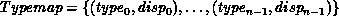Then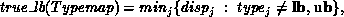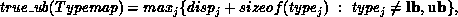and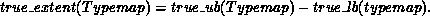(Readers should compare this with the definitions in Section 3.12.3 of the MPI-1 standard, which describes the function MPI_TYPE_EXTENT.)

The true_extent is the minimum number of bytes of memory necessary to hold a datatype, uncompressed.Up: New Datatype Manipulation Functions Next: Subarray Datatype Constructor Previous: Extent and Bounds of Datatypes